# Track Asset Depreciation with EZRentOut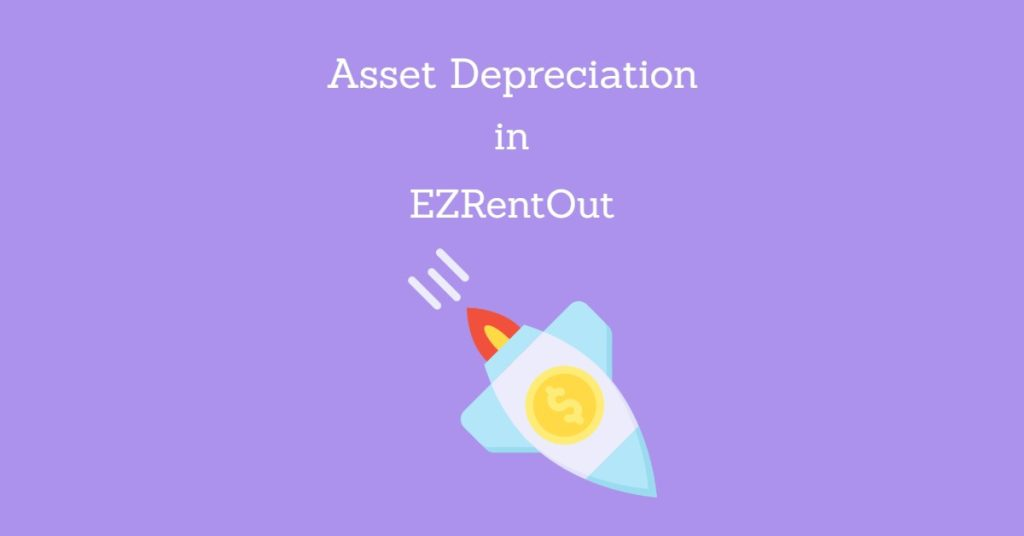Tracking asset depreciation is an essential business function and  EZRentOut makes it simple. Use the comprehensive asset depreciation functionality to track and manage depreciation across different asset groups, draft reports on that data and turn that data into actionable insights.

## 1. Getting started

There are two depreciation models available in EZRentOut, i.e. Straight Line method or Declining Balance method. You can either choose to depreciate assets on one model or you can even run both models at the same time. EZRentOut allows you to apply depreciation models at a group level. To enable this feature, go to Settings → Add Ons → Asset Depreciation → Enabled.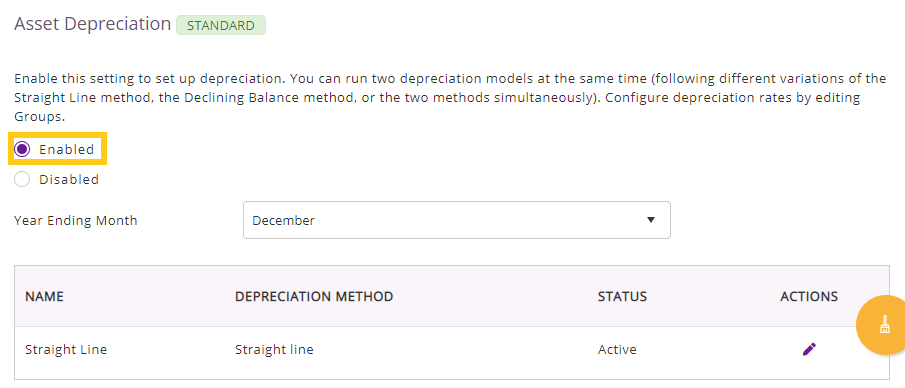The Straight Line depreciation method has been applied by default. To add the Declining Balance method, click on the button shown below: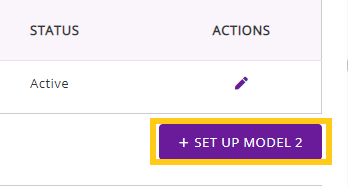This would open up a dialog box where you can choose ‘Declining Balance’, check on ‘Active’ and hit ‘Update’.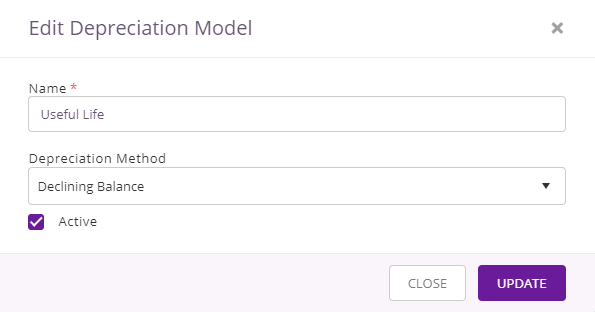You would see that the status of both methods will turn ‘Active’. If at any point, you want to deactivate a particular model, you can edit them by clicking on these buttons as follows: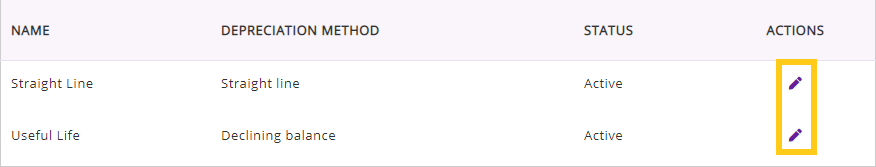Note: For the Declining Balance method, if you deactivate it and then Activate it again, you will lose all previous data on depreciation. The data you lose will not be recoverable.

Once activated both methods, go to Groups and choose the one you would like to set the depreciation rate for. You will see that the depreciation rate is set to 20% for your chosen method by default. As we have activated both methods, both are being applied on the Group and are shown with a depreciation rate of 20%.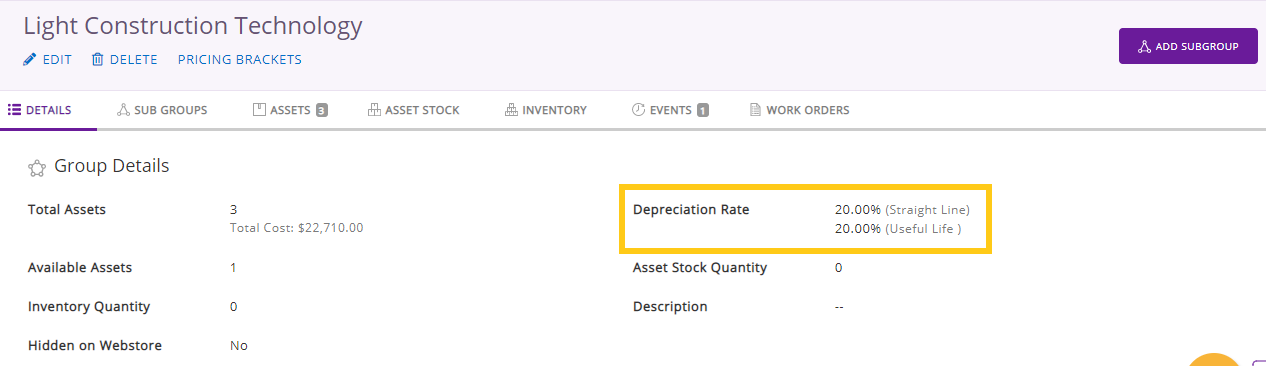To change the value for either method, click Edit, as shown below: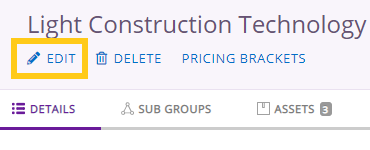This option allows you to change the depreciation rate as shown in the figure below: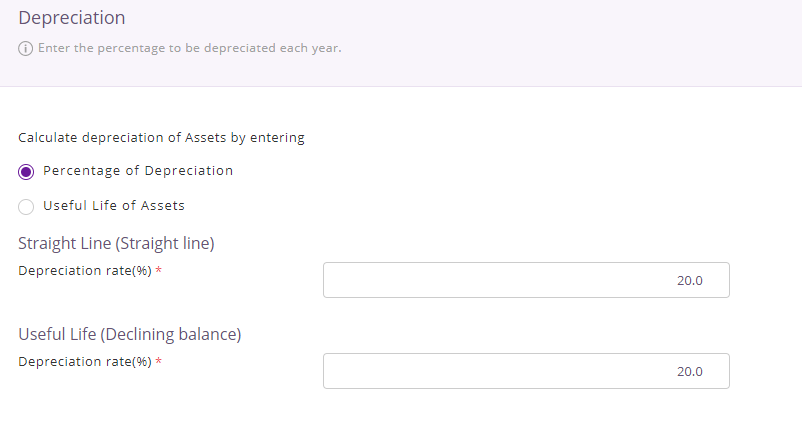Once you have edited the rates, click on ‘Update’ and the new rate will automatically be applied on the group.

## 2. How is depreciation calculated in EZRentOut?

Depreciation is calculated on a monthly basis in EZRentOut. The following examples will help you figure out how each method works:

### 2.1 Depreciation without a Salvage Value

Straight Line Method

Your company buys a hydraulic site dumper for \$3000 and sets the depreciation rate to 20%. The depreciation would be calculated as follows:

Monthly Depreciation

= [Purchase Cost * Depreciation Rate]/12

= [3000 * (20/100)]/12

= 600/12

=\$50.00

Let’s suppose that this specific asset was purchased on 1st January 2017 and you want to calculate its depreciated value on 1st July 2019 i.e., exactly 18 months after its procurement. As per Straight Line method, the value would be calculated in the following manner:

Depreciated Value After X Months

= Purchase Cost – [Monthly Depreciation * X]

= 3000 – [50 * 18]

= 3000 – 900

= \$2100

You can observe this mechanism here for an asset purchased on 4th February 2019, with the Heavy Construction Machinery group set to a depreciation rate of 20%.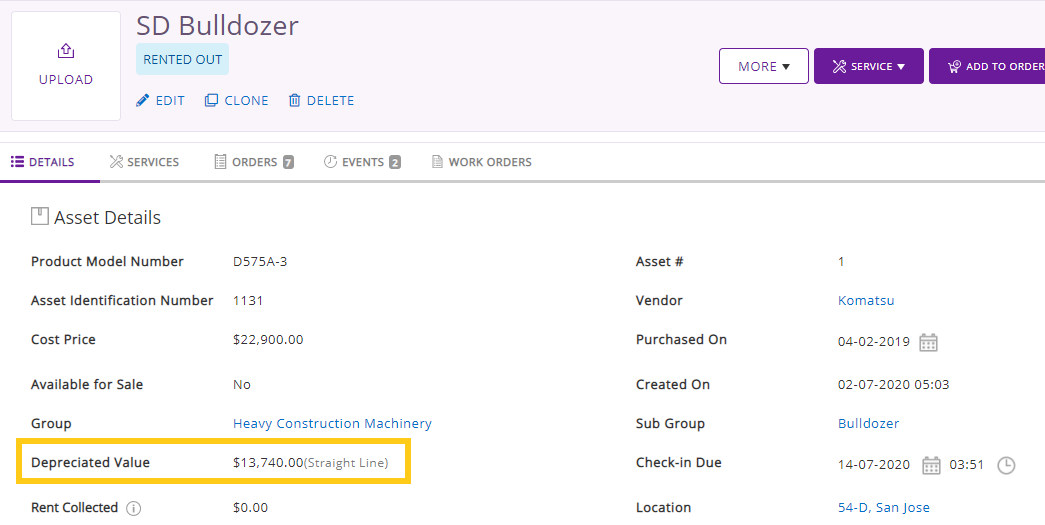Declining Balance Method

Now let’s suppose that your construction company is calculating depreciation using the declining balance method. The following scenario will help you better understand how this depreciation method works:

You own an SD Crawler Excavator worth \$500,000 purchased on 1st January 2017 and you set the depreciation rate as 40%. Here’s the calculation of depreciation expense and preparation of schedule:

 Year Book value of asset at the start of year Rate of declining balance Depreciation amount Book value of asset at the end of year 1 \$500,000 40% \$200,000 \$300,000 2 \$300,000 40% \$120,000 \$180,000 3 \$180,000 40% \$72,000 \$108,000 4 \$108,000 40% \$43,200 \$64,800 5 \$64,800 40% \$25,920 \$38,880 6 \$38,880 40% \$15,552 \$23,328 7 \$23,328 40% \$9,331 \$13,997 8 \$13,997 40% \$5,599 \$8,398 9 \$8,398 40% \$3,359 \$5,039 10 \$5,039 40% \$2,016 \$3,023

In this table, you can observe the depreciation schedule for the next 10 years from the date of purchase. This depreciation schedule will continue indefinitely for 20 years, after which the depreciated value will continue to be shown on the Asset Details page.

Note: When you are calculating depreciation using the declining balance method, you can edit the Purchase Date and Cost Price for the relevant asset during the first year of procurement but you cannot edit these fields when the first year ends.

Depreciated Value After X Months

If you want to calculate depreciation at a specific date, let’s say, September 2020, then it means that we are calculating the useful life for 8 months only. Here is how you can get the depreciated value:

= {[Annual depreciation expense / 12] * 8} – Book Value at start of year

= {[(108000 * 40%) / 12] * 8} – 108000

= 28800 – 108000

= \$79,200

### 2.2 Depreciation with a Salvage Value

Straight Line Method

The Salvage Value field is usually hidden in EZRentOut. However, you can still add this to your depreciation calculations. To enable the Salvage Value, go to Settings → Company Settings → Fields to Hide for Items. To be able to see this field, you will have to disable the setting as shown below: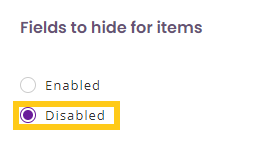To calculate depreciation, let’s consider the same example as shown above – a hydraulic site dumper for \$300 and a depreciation value of 20%. However, for this calculation, we will also consider adding a salvage value of \$100. You can add this by clicking Edit on the asset detail page. The depreciated value would be calculated as follows:

Monthly Depreciation

= [(Purchase Cost – Salvage Value) * Depreciation Rate]/12

= [(3000-100) * (20/100)]/12

= [(2900)*(0.2)]/12

= 580/12

= \$48.33

Now, let’s calculate the depreciated value after 12 months:

Depreciated Value After X Months

= Purchase Cost – [Monthly Depreciation * X]

= 3000 – [48.33 * 12]

= 3000 – 579.96

= \$2,420.04

Declining Balance Method

Now let’s consider another example to understand how depreciation with a salvage value will be calculated while using a declining balance method:

You own an SD Crawler Excavator worth \$500,000 purchased on 1st January 2016 and it has a useful life of 5 years with a depreciation rate of 40%. You have set the salvage value on \$50,000 after 5 years irrespective of its depreciation. Here is how you can calculate the depreciation expense and schedule for 5 years after purchasing the excavator:

 Year Book value of asset at the start of year Rate of declining balance Depreciation amount Book value of asset at the end of year 1 \$500,000 40% \$200,000 \$300,000 2 \$300,000 40% \$120,000 \$180,000 3 \$180,000 40% \$72,000 \$108,000 4 \$108,000 40% \$43,200 \$64,800 5 \$64,800 40% \$14,800 \$50,000

Now let’s study the \$14,800 highlighted value. The book value of SD Crawler Excavator at the beginning of year 5 is \$64,800. By multiplying it with the declining rate of 40%, you get \$25,920. If we keep on going with the same depreciation rate, then the book value of the machine at the end of year 5 will be \$38,880 (\$64,800 – \$25,920), which is less than the salvage value.

However, as we have set the salvage value at \$50,000 so the asset’s value cannot depreciate any further down with the declining balance method. Therefore, the depreciation in year 5 will be calculated as follows:

Book value at the beginning of the year – Salvage value =

\$64,800 – \$50,000 = \$14,800

Note: As of now, calculating the depreciation with salvage value has a limitation of 20 years. So, after 30 years, the salvage value will continue being shown as depreciation value for an indefinite period. If you want to calculate the depreciation value of an asset using this method, you will have to make sure that the asset purchase date is within the last 20 years.

## 3. Useful Life Depreciation Method

Another way through which you can calculate depreciation is through the lifespan of a particular asset. For example, if the recommended life for a particular asset is 10 years, then you can use either Declining Balance or Straight Line depreciation method for that useful life period. Given below are the steps for applying a useful life depreciation method.

Go to the Groups detail page. The Straight Line depreciation rate would be shown as follows: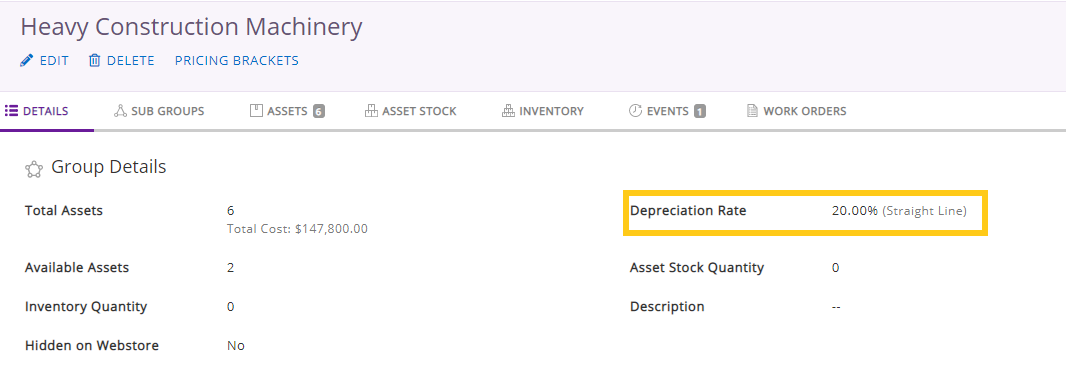Click on ‘Edit’ to change the type of depreciation. With this option, you can easily switch between Percentage of Depreciation and Useful Life of Assets. In our case, we will check ‘Useful Life of Assets’ as shown below: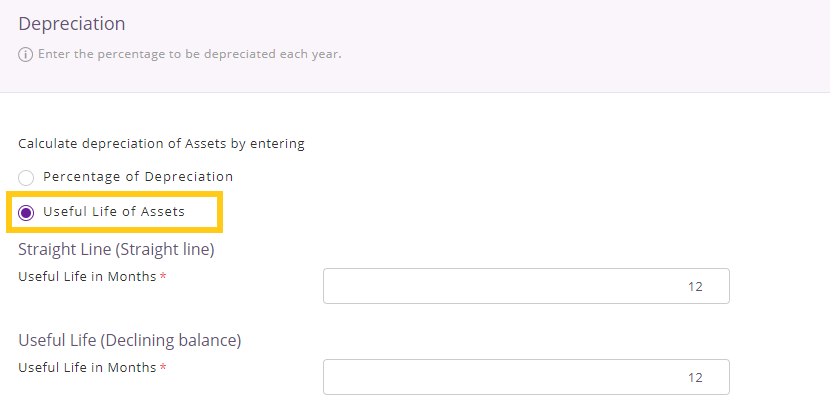Once you hit Update, it will show you the following dialog box cautioning you that switching to a different depreciation method will cause you to lose all previous data.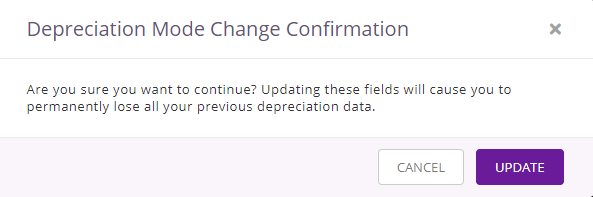Click on Update to reconfirm the changes.

Once the changes have been updated, you will be able to view all the changes regarding the depreciation mode under the ‘Events’ tab as shown below: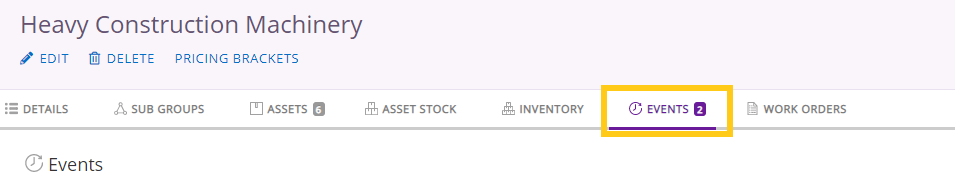## 4. Reports and analysis

You can also get reports for actionable insights on depreciation. To produce a report, go to Reports → Asset Reports → Depreciation. You will see the following filters: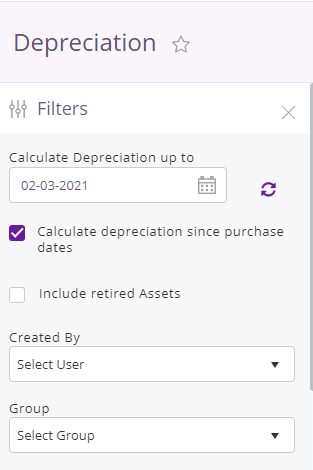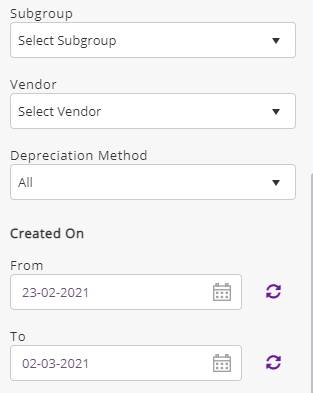Calculate Depreciation for These Dates: Depreciation will be calculated for the time period that you have selected. However, this option will only become visible when you uncheck the option to ‘Calculate Depreciation Since Purchase Date’.

Created by: You will be able to view the assets created by a specific User only.

Group: You will have to choose the Group for which you want the depreciation report to be drawn up for.

Subgroup: Similar to choosing a Group, you can also choose a Subgroup for which the depreciation report will be drawn up.

Vendor: It will show the assets supplied by the chosen vendor.

Created On: The assets created after a particular date will be shown.

Note: While drafting a report, if you pick an asset that has a Purchase Date later than the date you’re attempting to calculate depreciation from, then the system will revert the date as the starting point of depreciation calculation.

If you want to include Retired Assets in your report, you can do so by calculating the depreciation since the purchase dates of those assets.

If you have activated both depreciation models, then the report will also show an added filter through which you can further narrow down the results.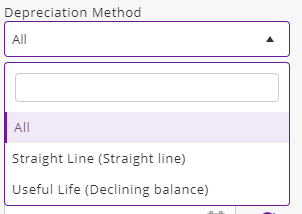## 5. Depreciation during the Purchase, Disposal and Retiring Month

On Purchase: During the first month of an asset’s life, EZRentOut uses Full-Month Depreciation convention – irrespective of when it was purchased during the month – it kicks in on the last day of the next month. Therefore, where depreciation is concerned, you can buy the asset on the 7th of January or 27th of January, it would not make any difference to the depreciation being calculated as they would both be depreciated on 28th of February.

The updated depreciated value can be viewed on the relevant asset’s detail page.

On Disposal: EZRentOut doesn’t charge depreciation for the last month of an asset’s life no matter when it is disposed of. As the depreciation of an asset is charged by the end of the next month, there is no ‘next month’ in this scenario and hence, no depreciation is charged.

Note: In EZRentOut, depreciation is charged before the User retires an asset. This means that if an item is retired on 1st December, then depreciation will be charged for November and then the item will be retired.

On Retiring: When an asset is retired, the depreciated value field keeps on showing up on the asset detail page. However, if you have added a salvage value for that particular asset, then the depreciated value field will keep on showing the salvage value even when the asset is retired.

## 6. Setting your Year Ending Month

For calculating depreciation, you can also choose the year ending month by clicking on Settings → Add Ons → Year Ending Month. You will see the following screen: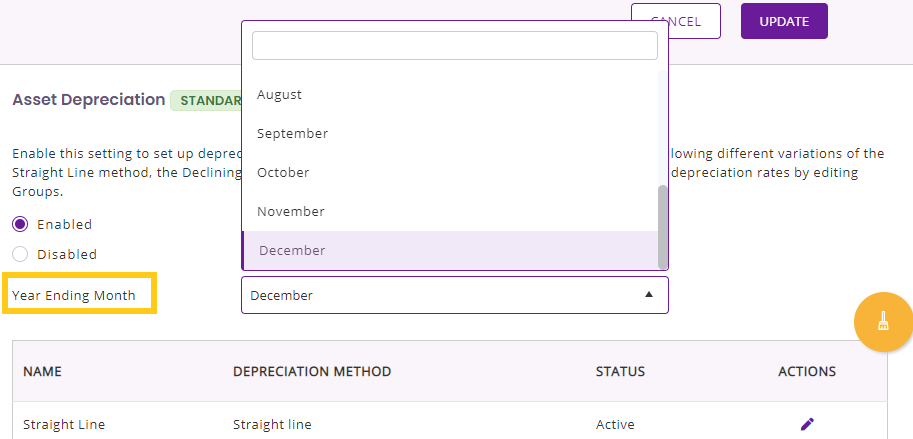Suppose that you have chosen June as your year ending month. You buy an asset on 1st March 2020 and your year is ending on 31st June 2020. So, in this scenario, the depreciation will be calculated for only three months and declining balance method will be used.

Note: You cannot change the year ending month once the calculation for depreciation has already been done.